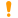# Factorial

 Block Group: Math Operations Icon:The Factorial block returns the single factorial of a number. The single factorial of n is equal to 1*2*3*…* n.

For information on using dataflow blocks, see Dataflow.

For accepted and excluded arguments of Math Operations blocks, see Math Operations.

## Input/Output Property

The following property of the Factorial block can take input and give output.

• input (number)

input specifies the number to find the factorial for.

## Output Property

The following property of the Factorial block can give output but cannot take input.

• output (number)

output returns the factorial of the input.

## Special Cases for the Factorial Block

The following are special cases for the Factorial block:

• A negative input value causes 1 to be returned.
• If the input value is not an integer, it is truncated.
• The factorial of zero is one.
• Error values, and text that cannot be resolved to a number, cause 1 to be returned.

## Examples

The following image shows four examples of the Factorial block.